# Modulation Index or Modulation Factor of AM Wave

## Modulation Index or Modulation Factor

In AM wave, the modulation index (m) is defined as the ratio of amplitudes of the modulating signal to the amplitude of carrier signal. i.e. ,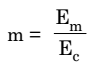Where Eis the amplitude of modulating signal

Ec  is the amplitude of carrier signal

When Em ≤ E, the modulation index ‘m’ has values between 0 and 1 and no distortion is introduced in the AM wave.

But, if Em > E, then m is greater than 1. This will distort the shape of AM signal. The distortion is called as over modulation.

The modulation index is also called as modulation factor, modulation coefficient or degree of modulation.

However, this modulation index is expressed as percentage and it is called as percentage modulation. Therefore,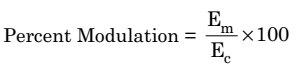Here, m is a dimensionless quantity.

Depending on the value of percentage modulation (m), the AM wave may be classified into following two categories:

1. Linear modulation
2. Over modulation

### Linear Modulation :

If m ≤ 1 or if the percentage modulation is less than 100, then the type of modulation is linear amplitude modulation.

The waveforms of AM waves with linear modulation have been shown in figures 2 and 3 respectively .

### Over Modulation :

If m > 1, i.e., if the percentage modulation is greater than 100%, then the type of modulation is called as  overmodulation.

Since m > 1, the envelope can sometimes reverse the phase as shown in figure 4.

Overmodulation introduces envelope distortion. Therefore, it should be avoided.

### Example 1.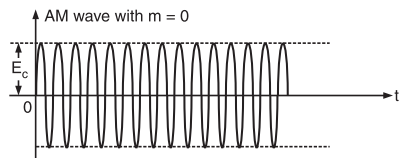Fig. 1: AM Wave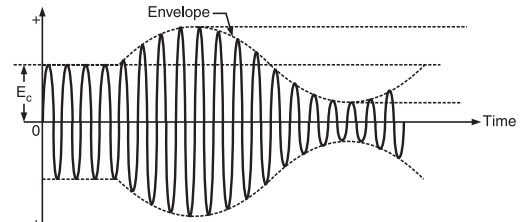Fig. 2 : AM Wave for percentage modulation less than 100%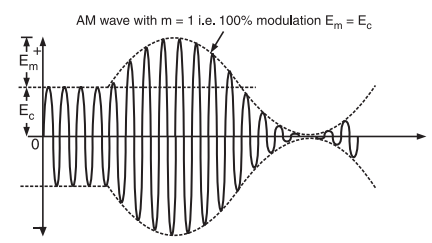Fig. 3 : AM Wave with percentage modulation =100%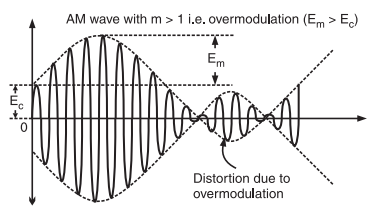Fig. 4 : AM Wave with over modulation

## Evaluation of  Modulation Index

In practice, the modulation index can be measured using the following two methods:

(i) Using the AM signal as it is.

(ii) Using the trapezoidal display of the AM wave.

### Modulation Index Evaluation using the AM Wave

If the AM signal is displayed on the screen of a cathode ray oscilloscope (CRO), it appears to be as shown in figure 5.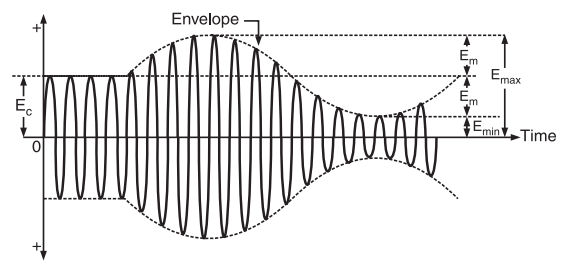Fig. 5 : AM Wave for calculation of m

The x-axis represents the time and y-axis represents the amplitude in volts.The dotted line which represents the envelope of AM signal does not actually appear on the screen.

In order to calculate modulation index m which iswe must express Em and Ec in terms of Emax and Emin.

From figure 5, we can write,

and Ec = Emax – E…………………….(2)

Substituting the value of Efrom equation (1) into equation (2), we get,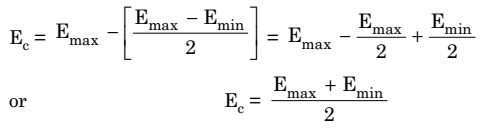…………………(3)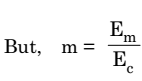Now, substituting the values of Em and Efrom equations (1) and (3), we get,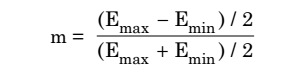Hence,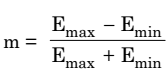This is the required expression.

### Evaluation of Modulation Index using Trapezoidal Display

The set up for evaluating the modulation index using trapezoidal display is shown in figure 6 and the corresponding waveforms are shown in figure 7.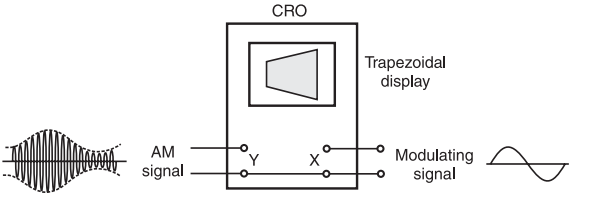Fig.6 : Setup for evaluating modulation index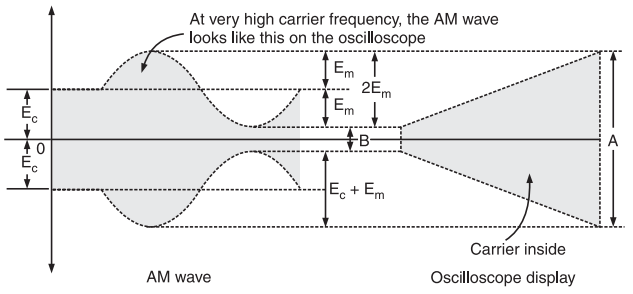Fig.7 : Trapezoidal display of AM signal

In this method, the AM signal is connected to vertical deflection plates of the oscilloscope and the modulating signal is applied to the horizontal deflection plates of the oscilloscope.

The display on the screen of the oscilloscope is of trapezoidal shape as shown in figure 7.

From figure 7, we can write,

A = 2 (Ec + Em) ………………….(4)

and

B = 2 (Ec – Em) ……………………… (5)

Therefore, adding equations (4) and (5), we get,

A + B = 4 Ec

Or,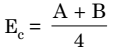………………….. (6)

Now, subtracting  equations (5) from equation (4) , we get ,

A -B = 4 Em

Or,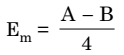…………………. (7)

The modulation index,Substituting the values of Em and Ec, we get,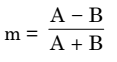Thus, we can get the modulation index by measuring the length A and B.